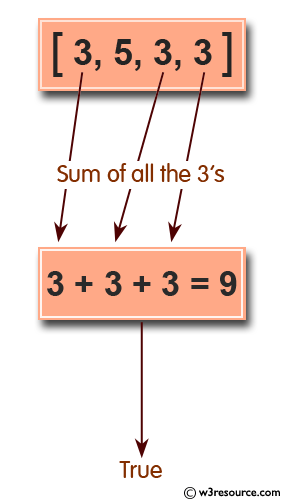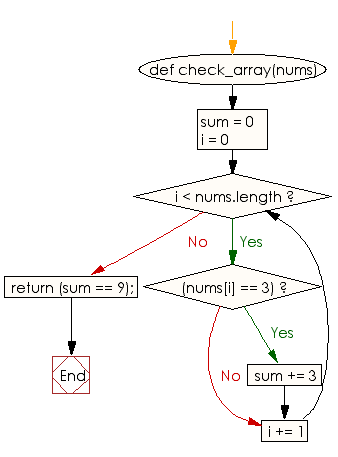﻿ Ruby Array exercises: Check whether the sum of all the 3's of an given array of integers is exactly 9 - w3resource# Ruby Array Exercises: Check whether the sum of all the 3's of an given array of integers is exactly 9

## Ruby Array: Exercise-33 with Solution

Write a Ruby program to check whether the sum of all the 3's of an given array of integers is exactly 9.Ruby Code:

``````def check_array(nums)
sum = 0
i = 0
while i < nums.length
if(nums[i] == 3)
sum += 3
end
i += 1
end
return (sum == 9);
end
print check_array([3, 5, 3, 3]),"\n"
print check_array([3, 3, 2, 1]),"\n"
print check_array([3, 3, 3, 3]),"\n"
``````

Output:

```true
false
false
```

Flowchart:Ruby Code Editor: